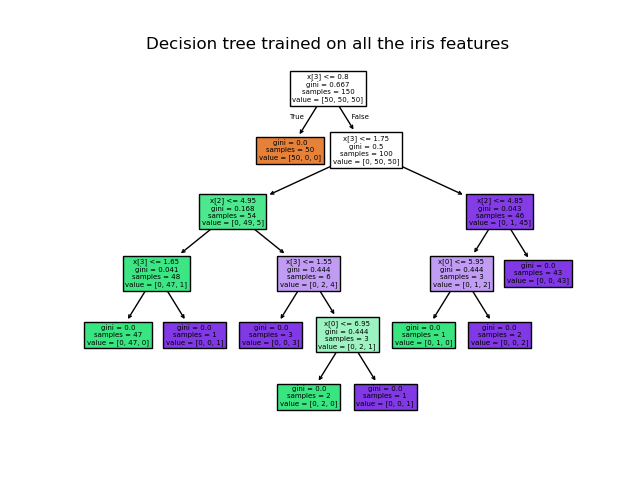# Plot the decision surface of decision trees trained on the iris dataset¶

Plot the decision surface of a decision tree trained on pairs of features of the iris dataset.

For each pair of iris features, the decision tree learns decision boundaries made of combinations of simple thresholding rules inferred from the training samples.

We also show the tree structure of a model built on all of the features.

First load the copy of the Iris dataset shipped with scikit-learn:

from sklearn.datasets import load_iris



Display the decision functions of trees trained on all pairs of features.

import numpy as np
import matplotlib.pyplot as plt

from sklearn.tree import DecisionTreeClassifier
from sklearn.inspection import DecisionBoundaryDisplay

# Parameters
n_classes = 3
plot_colors = "ryb"
plot_step = 0.02

for pairidx, pair in enumerate([[0, 1], [0, 2], [0, 3], [1, 2], [1, 3], [2, 3]]):
# We only take the two corresponding features
X = iris.data[:, pair]
y = iris.target

# Train
clf = DecisionTreeClassifier().fit(X, y)

# Plot the decision boundary
ax = plt.subplot(2, 3, pairidx + 1)
DecisionBoundaryDisplay.from_estimator(
clf,
X,
cmap=plt.cm.RdYlBu,
response_method="predict",
ax=ax,
xlabel=iris.feature_names[pair],
ylabel=iris.feature_names[pair],
)

# Plot the training points
for i, color in zip(range(n_classes), plot_colors):
idx = np.where(y == i)
plt.scatter(
X[idx, 0],
X[idx, 1],
c=color,
label=iris.target_names[i],
cmap=plt.cm.RdYlBu,
edgecolor="black",
s=15,
)

plt.suptitle("Decision surface of decision trees trained on pairs of features")
_ = plt.axis("tight")/home/circleci/project/examples/tree/plot_iris_dtc.py:64: UserWarning:

No data for colormapping provided via 'c'. Parameters 'cmap' will be ignored

/home/circleci/project/examples/tree/plot_iris_dtc.py:64: UserWarning:

No data for colormapping provided via 'c'. Parameters 'cmap' will be ignored

/home/circleci/project/examples/tree/plot_iris_dtc.py:64: UserWarning:

No data for colormapping provided via 'c'. Parameters 'cmap' will be ignored

/home/circleci/project/examples/tree/plot_iris_dtc.py:64: UserWarning:

No data for colormapping provided via 'c'. Parameters 'cmap' will be ignored

/home/circleci/project/examples/tree/plot_iris_dtc.py:64: UserWarning:

No data for colormapping provided via 'c'. Parameters 'cmap' will be ignored

/home/circleci/project/examples/tree/plot_iris_dtc.py:64: UserWarning:

No data for colormapping provided via 'c'. Parameters 'cmap' will be ignored


Display the structure of a single decision tree trained on all the features together.

from sklearn.tree import plot_tree

plt.figure()
clf = DecisionTreeClassifier().fit(iris.data, iris.target)
plot_tree(clf, filled=True)
plt.title("Decision tree trained on all the iris features")
plt.show()Total running time of the script: ( 0 minutes 0.803 seconds)

Gallery generated by Sphinx-Gallery